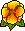## Weighted Regularization of Maxwell Equations -- Computations in Curvilinear Polygons

Martin Costabel, Monique Dauge, Daniel Martin, Gregory VialWe present numerical experiments using the method of regularizing time harmonic Maxwell equations by a divergence part adapted to the singular geometry of the domain, see the Paper for the theory. This method is applied to polygonal domains with straight or curvilinear edges, and reentrant corners. The Galerkin discretization uses geometrical refined meshes and ``high" degree polynomial (of degree ranging from 1 to 10). With this, we reach a relative error of 10 to -8 for the first TE eigenvalue with a little bit more than 10000 unknowns (note that the corresponding eigenvector is highly singular - unbounded). The convergence rates which we obtain evoque an exponential convergence rate.16 December 2001.

For the Proceedings of Enumath'01 in Ischia, ItalyPDF file (132 k)